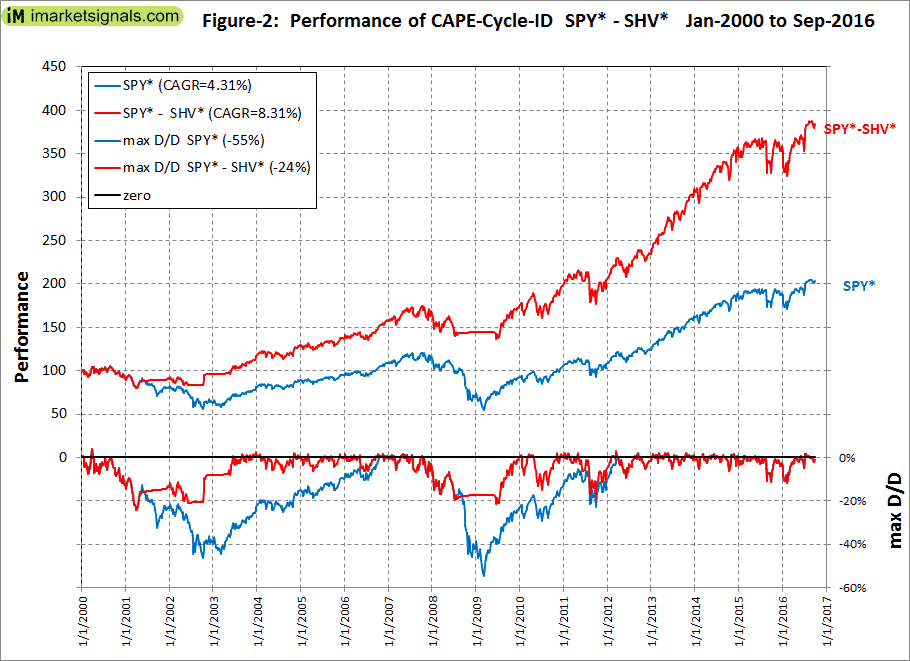## Tajma börsen med hjälp av Schillers CAPE

The construction and rules for our CAPE-Cycle-ID model are summarized below. The rules differ from the Wong model only in respect to the investment choices and trading times.

##### Construction:
1. A primary oscillator is constructed from the year-over-year rate-of-change of the CAPE (YoY-ROC-CAPE).
2. The exponential moving average (EMA) of the YoY-ROC-CAPE is then calculated with a smoothing factor of 0.20 (EMA-YoY-ROC-CAPE).
3. A secondary indicator is calculated from the month-over-month change of the EMA-YoY-ROC-CAPE (MoM-EMA-YoY-ROC-CAPE).
##### Rules:
1. Score +1 when the EMA-YoY-ROC-CAPE is greater or equal to -13%, otherwise score -1.
2. Score +1 when the MoM-EMA-YoY-ROC-CAPE is greater than zero or when it has a positive slope, i.e. when the current month level is greater than the previous month level, otherwise score -1.
3. Sum the scores for each month. Possible combined scores are +2, 0, and -2.
4. Invest in the stock market when the combined score is +2 or 0.
5. Go to cash or bonds when the combined score is -2.